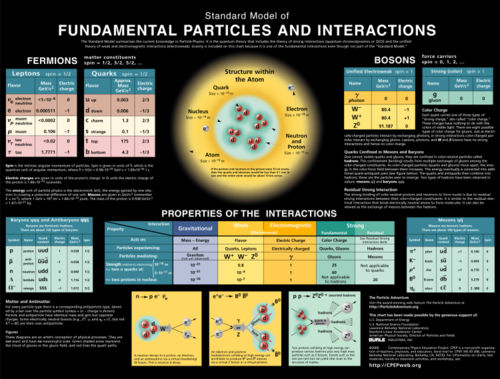## What is Particle Physics?

Particle physics is the study of the universe at its most fundamental level: the elementary particles which are the basic building blocks of matter, and the forces which allow them to interact.

Our current understanding of particle physics is summarized in the Standard Model.In the Standard Model, all matter is made of fermions (quarks, like the "up" and "down" quarks that make up protons and neutrons, and leptons, like the electron). All forces are carried by gauge bosons: the photon carries the electromagnetic force, the W and Z bosons carry the weak force (responsible for nuclear beta decay) and the gluons carry the strong force (which holds the nuclei of atoms together). Gravity is the only known force not included in the Standard Model, as it is much weaker than the other forces at the scales probed by current experiments (gravity holds you on the Earth, so you notice it, but it does not even begin to pull apart the atoms in your body, which are firmly held together by the other forces).

Physicists hope that by doing experiments at higher energies, we will some day be able to understand how gravity fits in with the other forces. We also want to understand why the fermions have the masses that they do, and what makes up all the dark matter in the universe. Experiments at the LHC will help us to answer these questions.